# Preface

This is a brief example report using `dataquieR`’s functions. For a longer and better elaborated example, please also consider our online example with data from SHIP.

# INTEGRITY

## Study data

``````load(system.file("extdata", "study_data.RData", package = "dataquieR"))
sd1 <- study_data``````

The imported study data consist of:

• N = 3000 observations and
• P = 53 study variables

``````load(system.file("extdata", "meta_data.RData", package = "dataquieR"))
md1 <- meta_data``````

The imported meta data provide information for:

• P = 53 study variables and
• Q = 20 attributes

## Applicability

The call of this R-function requires two inputs only:

``````appmatrix <- pro_applicability_matrix(study_data = sd1,
meta_data = md1,
label_col = LABEL)``````

Heatmap-like plot:

``appmatrix\$ApplicabilityPlot``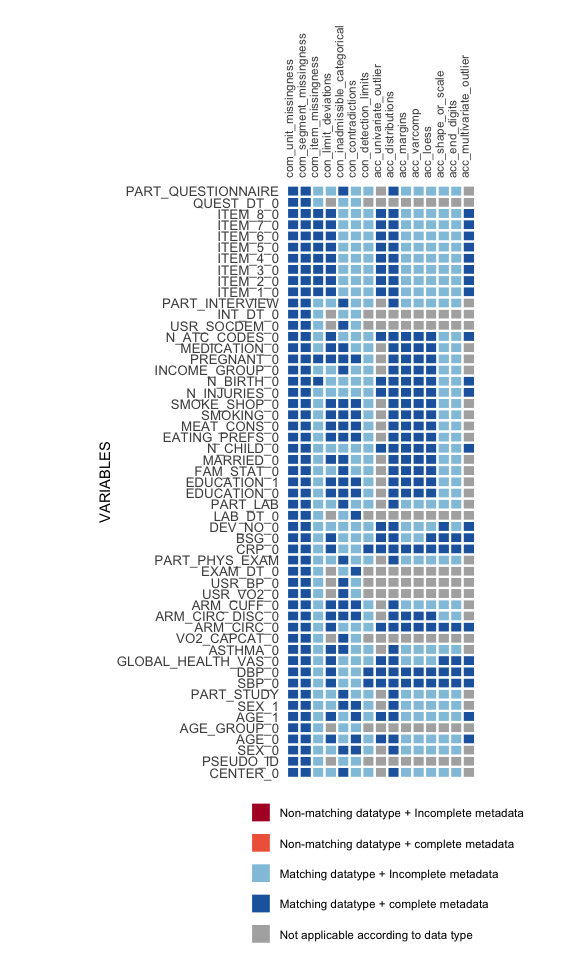# COMPLETENESS

## Unit missingness

``````my_unit_missings2 <- com_unit_missingness(study_data  = sd1,
meta_data   = md1,
id_vars     = c("CENTER_0", "PSEUDO_ID"),
strata_vars = "CENTER_0",
label_col   = "LABEL")``````
``my_unit_missings2\$SummaryData``

## Segment missingness

``````MissSegs <- com_segment_missingness(study_data = sd1,
meta_data = md1,
label_col = "LABEL",
threshold_value = 5,
direction = "high",
exclude_roles = c("secondary", "process"))``````
``MissSegs\$SummaryPlot``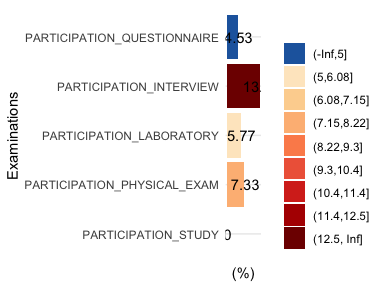For some analyses adding new and transformed variable to the study data is necessary.

``````# use the month function of the lubridate package to extract month of exam date
require(lubridate)
# apply changes to copy of data
sd2 <- sd1
# indicate first/second half year
sd2\$month <- month(sd2\$v00013)``````

``````MD_TMP <- prep_add_to_meta(VAR_NAMES    = "month",
DATA_TYPE    = "integer",
LABEL        = "EXAM_MONTH",
VALUE_LABELS = "1 = January | 2 = February | 3 = March |
4 = April | 5 = May | 6 = June | 7 = July |
8 = August | 9 = September | 10 = October |
11 = November | 12 = December",
meta_data    = md1)``````

Subsequent call of the R-function may include the new variable.

``````MissSegs <- com_segment_missingness(study_data = sd2,
meta_data = MD_TMP,
group_vars = "EXAM_MONTH",
label_col = "LABEL",
threshold_value = 1,
direction = "high",
exclude_roles = c("secondary", "process"))``````
``MissSegs\$SummaryPlot``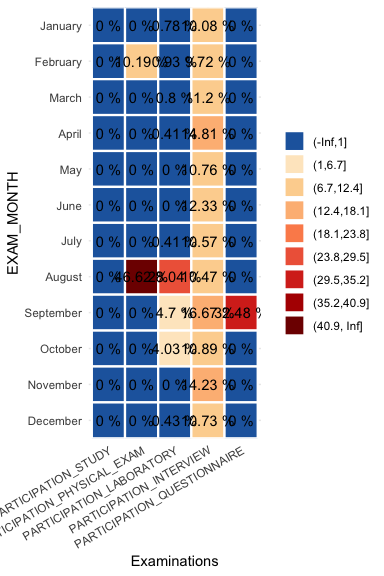## Item missingness

The following implementation considers also labeled missing codes. The use of such a table is optional but recommended. Missing code labels used in the simulated study data are loaded as follows:

``````code_labels <- read.csv2(system.file("extdata",
"Missing-Codes-2020.csv",
package = "dataquieR"),
stringsAsFactors = FALSE, na.strings = c())``````
``````item_miss <- com_item_missingness(study_data      = sd1,
meta_data       = meta_data,
label_col       = 'LABEL',
show_causes     = TRUE,
cause_label_df  = code_labels,
include_sysmiss = TRUE,
threshold_value = 80
) ``````

The function call above sets the analyses of causes for missing values to TRUE, includes system missings with an own code, and sets the threshold to 80%.

``item_miss\$SummaryTable``

#### Summary plot of item missingness

``item_miss\$SummaryPlot``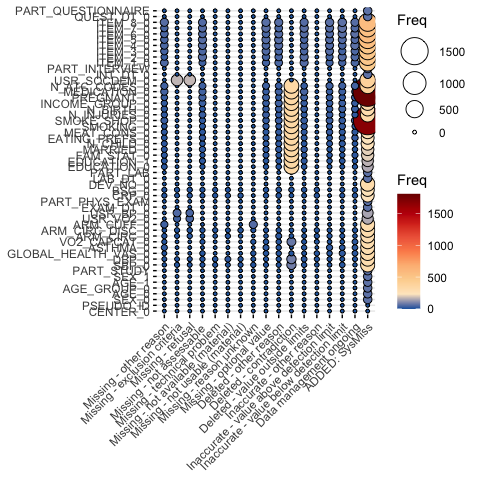# CONSISTENCY

## Limit deviations

``````MyValueLimits <- con_limit_deviations(resp_vars  = NULL,
label_col  = "LABEL",
study_data = sd1,
meta_data  = md1,
limits     = "HARD_LIMITS")``````

### Summary table

``MyValueLimits\$SummaryTable``

### Summary plot

``````# select variables with deviations
``ggpubr::ggarrange(plotlist = MyValueLimits\$SummaryPlotList[whichdeviate], ncol = 2) ``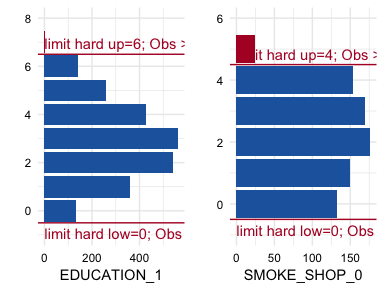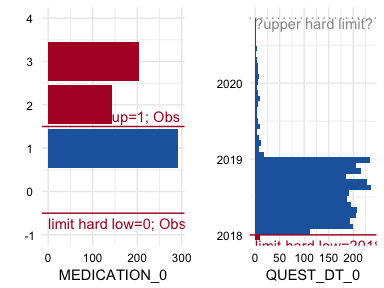``````IAVCatAll <- con_inadmissible_categorical(study_data = sd1,
meta_data  = md1,
label_col  = "LABEL")``````

``````checks <- read.csv(system.file("extdata",
``````AnyContradictions <- con_contradictions(study_data      = sd1,
``AnyContradictions\$SummaryTable``
``AnyContradictions\$SummaryPlot ``